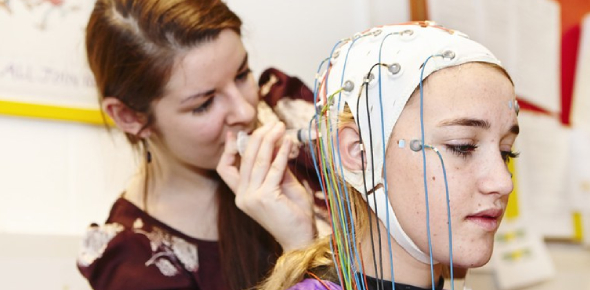# Can You Pass This Experimental Psychology Test?

10 Questions | Total Attempts: 2584SettingsThe Experimental Psychology analyses relationships between human behaviour and the mind. It is centred on fact-based and scientific research. This quiz has been designed to test your initial understanding of the field of experimental psychology and will allow you to learn about your base knowledge for the preparation of the exams. So, let's try out the quiz. All the best!

• 1.
Who is the Father of Experimental Psychology?
• A.

Sigmund Freud

• B.

Abraham Maslow

• C.

Hermann Ebbinghaus

• D.

Wilhelm Wundt

• 2.
• A.

Testing correlational hypothesis

• B.

Validating hypothetical situations

• C.

Knowing the significant effect of a therapy

• D.

Identifying causal relationship

• 3.
It the most stage of a study that is prerequisite to an experimental study.
• A.

Correlation

• B.

Regression studies

• C.

Structure studies

• D.

Exploratory relationship

• 4.
A research hypothesis is considered experimental in nature when it assesses.
• A.

Difference

• B.

Relationship

• C.

Structure

• D.

Prediction

• 5.
In doing experimental studies, researchers should assure that respondents are provided with complete knowledge of the impact of the experiment on them. It is called.
• A.

Confidentiality

• B.

Debriefing

• C.

Informed consent

• D.

Double blind

• 6.
In an experiment, the variable manipulated by the experimenter is the ________ variable, and the behaviour recorded by the experimenter is the ________ variable
• A.

Observed; control

• B.

Independent; dependent

• C.

Dependent; independent

• D.

Control; observed

• 7.
Experiments are conducted in order to:
• A.

All of these

• B.

Replicate and extend previous findings

• C.

Just see what happens.

• D.

Test theories

• 8.
In a between-subjects experimental design, each participant is given ________ level of the independent variable, whereas, in a within-subjects design, each participant is given ________ level of the independent variable.
• A.

One; each

• B.

One; one

• C.

Each; one

• D.

Each; each

• 9.
Quasi-experiments differ from true experiments in that quasi-experiments involve:
• A.

Naturally occurring dependent variables.

• B.

Using only animal participants.

• C.

Naturally occurring independent variables

• D.

Greater demand characteristics.

• 10.
Psychologists attempt to make different groups of participants as similar as possible via:
• A.

Randomization

• B.

Confounding

• C.

Correlation

• D.

Regression to the mean

Related TopicsBack to top